# Find Circles and Ellipses in an Image using OpenCV | Python

To identify circles, ellipses, or in general, any shape in which the pixels are connected we use the SimpleBlobDetector() function of OpenCV. In non-technical terms, a blob is understood as a thick liquid drop. Here, we are going to call all shapes a blob. Our task is to detect and recognize whether the blob is a circle or not.

OpenCV provides a convenient way to detect blobs and filter them based on different characteristics. There are various different parameters that control the identification process and the results. The important parameters used for this project are:

• Filter by Area – This is to avoid any identification of any small dots present in the image that can be wrongly detected as a circle.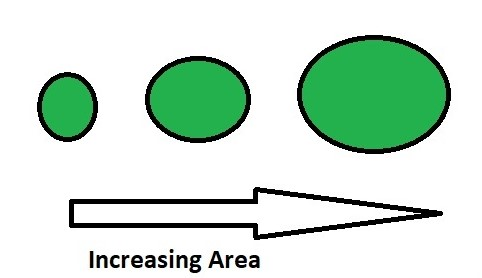• Filter by Circularity – This helps us to identify, shapes that are more similar to a circle.
`Circularity =. `

A true circle has a circularity of 1, a square has a circularity near 78%.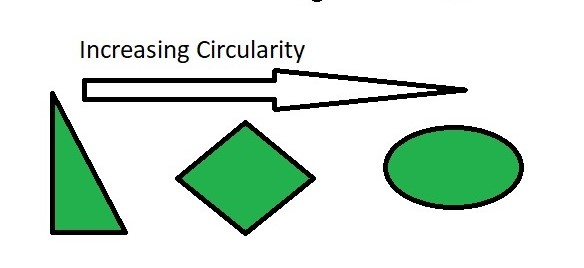• Filter by Convexity – Concavity in general, destroys the circularity. More is the convexity, the closer it is to a close circle.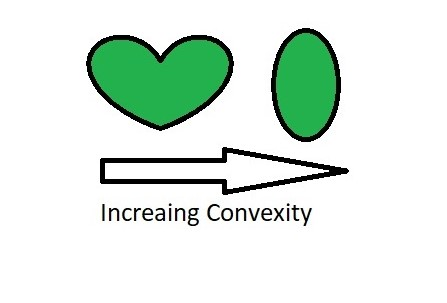• Filter by Inertia – Objects similar to a circle has larger inertial.E.g. for a circle, this value is 1, for an ellipse it is between 0 and 1, and for a line it is 0. To filter by inertia ratio, set filterByInertia = 1, and set, 0 <= minInertiaRatio <= 1 and maxInertiaRatio (<=1 ) appropriately.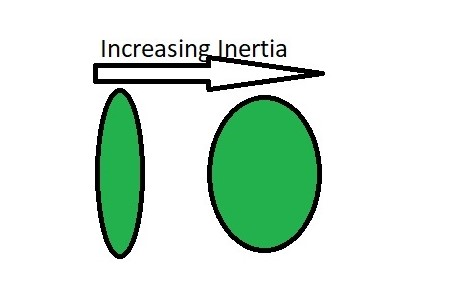Below is the code for identifying Circles:

## Python3

 `import` `cv2 ` `import` `numpy as np ` ` `  `# Load image ` `image ``=` `cv2.imread(``'C://gfg//images//blobs.jpg'``, ``0``) ` ` `  `# Set our filtering parameters ` `# Initialize parameter setting using cv2.SimpleBlobDetector ` `params ``=` `cv2.SimpleBlobDetector_Params() ` ` `  `# Set Area filtering parameters ` `params.filterByArea ``=` `True` `params.minArea ``=` `100` ` `  `# Set Circularity filtering parameters ` `params.filterByCircularity ``=` `True`  `params.minCircularity ``=` `0.9` ` `  `# Set Convexity filtering parameters ` `params.filterByConvexity ``=` `True` `params.minConvexity ``=` `0.2` `     `  `# Set inertia filtering parameters ` `params.filterByInertia ``=` `True` `params.minInertiaRatio ``=` `0.01` ` `  `# Create a detector with the parameters ` `detector ``=` `cv2.SimpleBlobDetector_create(params) ` `     `  `# Detect blobs ` `keypoints ``=` `detector.detect(image) ` ` `  `# Draw blobs on our image as red circles ` `blank ``=` `np.zeros((``1``, ``1``))  ` `blobs ``=` `cv2.drawKeypoints(image, keypoints, blank, (``0``, ``0``, ``255``), ` `                          ``cv2.DRAW_MATCHES_FLAGS_DRAW_RICH_KEYPOINTS) ` ` `  `number_of_blobs ``=` `len``(keypoints) ` `text ``=` `"Number of Circular Blobs: "` `+` `str``(``len``(keypoints)) ` `cv2.putText(blobs, text, (``20``, ``550``), ` `            ``cv2.FONT_HERSHEY_SIMPLEX, ``1``, (``0``, ``100``, ``255``), ``2``) ` ` `  `# Show blobs ` `cv2.imshow(``"Filtering Circular Blobs Only"``, blobs) ` `cv2.waitKey(``0``) ` `cv2.destroyAllWindows() `

Output: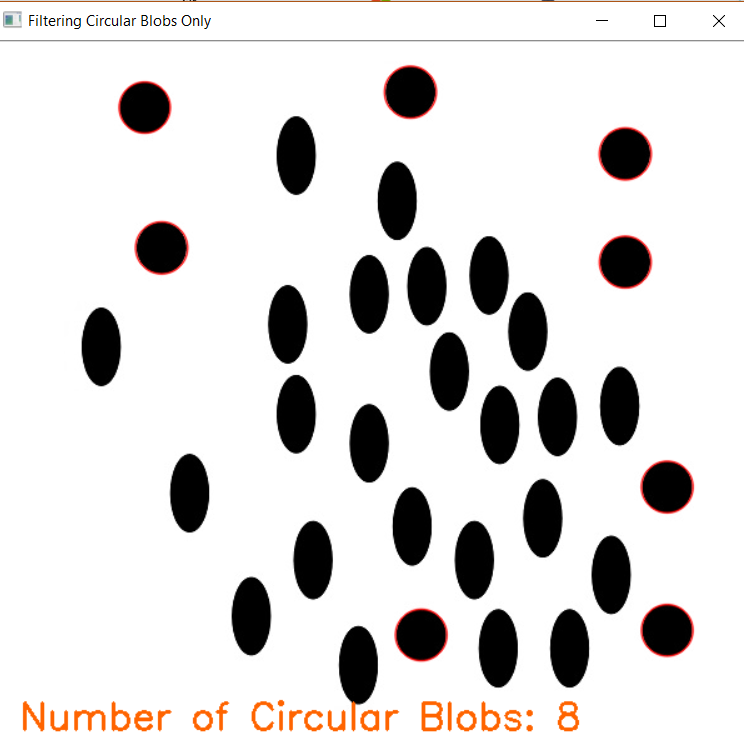Whether you're preparing for your first job interview or aiming to upskill in this ever-evolving tech landscape, GeeksforGeeks Courses are your key to success. We provide top-quality content at affordable prices, all geared towards accelerating your growth in a time-bound manner. Join the millions we've already empowered, and we're here to do the same for you. Don't miss out - check it out now!